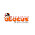## Friday, December 12, 2014

### December 2014 / January 2015 Newsflash: Tips & Techniques

Upper Elementary and Middle School

Find the perimeter of the shape below. (Hint: there is enough information.)

Solution:

The length on the bottom of the rectangle is the same as the length on the top, although the top has been split into two pieces. Similarly the width on the left is 11 inches and the width on right is also 11 inches, again in two pieces.

Now, the length of the side is given in inches and the length of the bottom is given in feet. Since we have to add to get the perimeter, the Law of SAMEness requires the units to be the same. Let’s change 2 feet into 24 inches (2 x 12).

Note that it is not necessary to know the exact measurement of the length and width. All that matters is that we know the total length of each.

With that, 11 in + 11 in + 24 in + 24 in = 70 inches.

Algebra

If ax + 2a = c and x + 2 = 3, express a in terms of c

Solution

If ax + 2a = c, then a(x + 2) = c. Now, substitute “3” for “x + 2” and you get 3a = c
so a = c/3.

1.2.